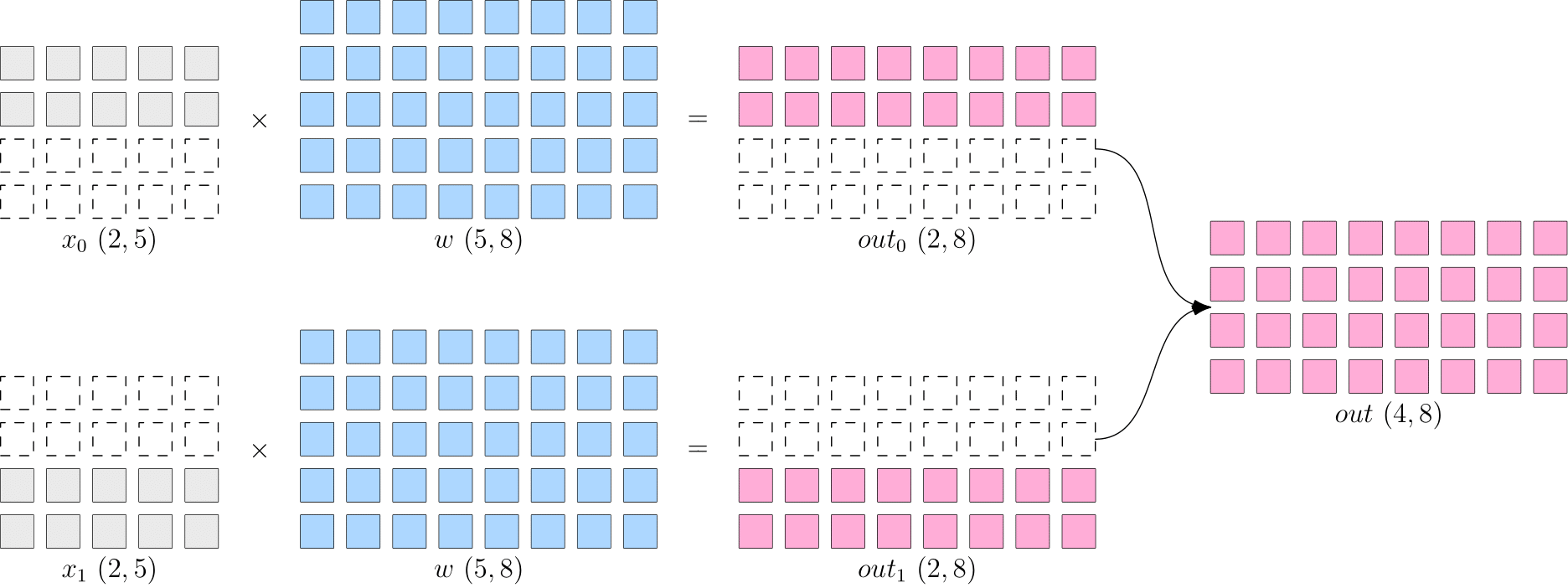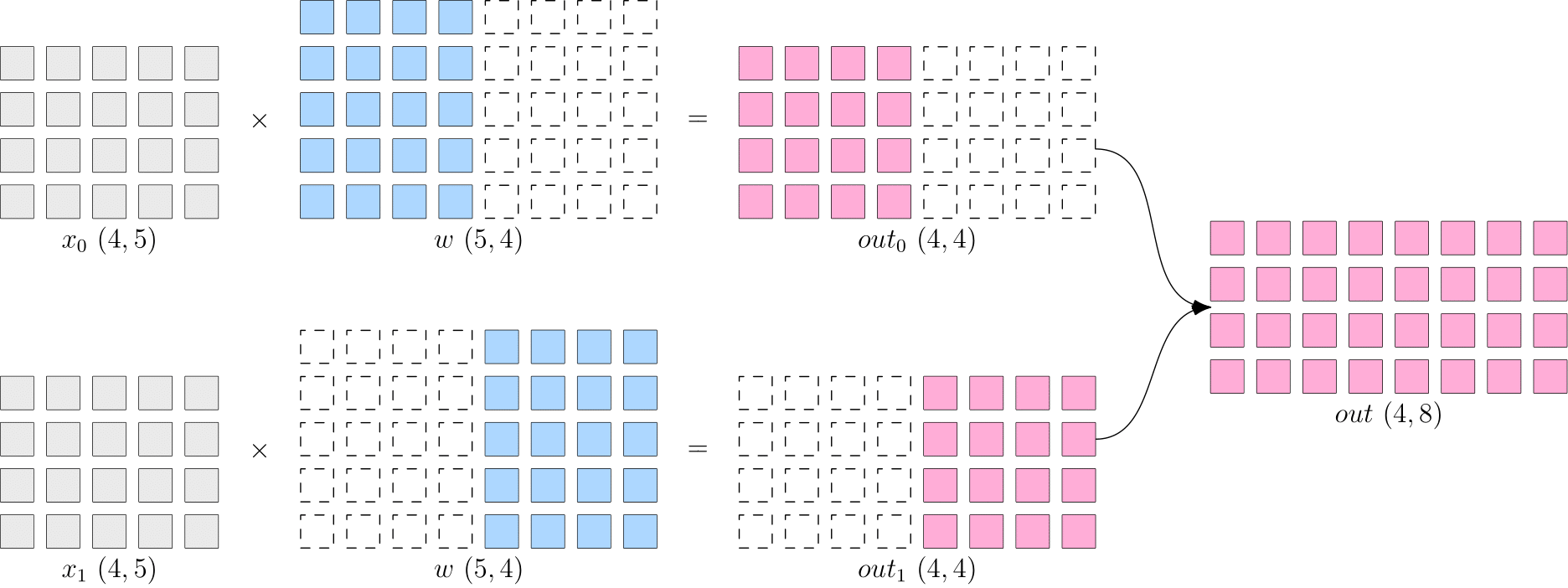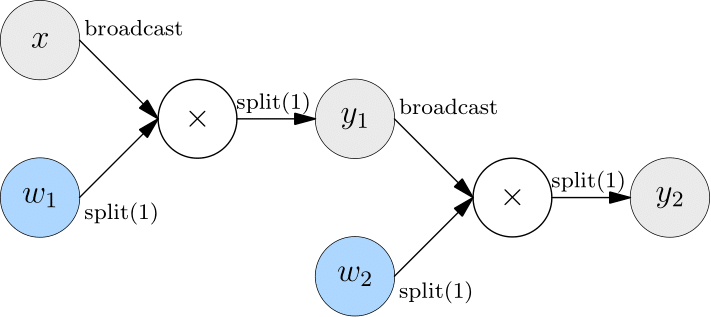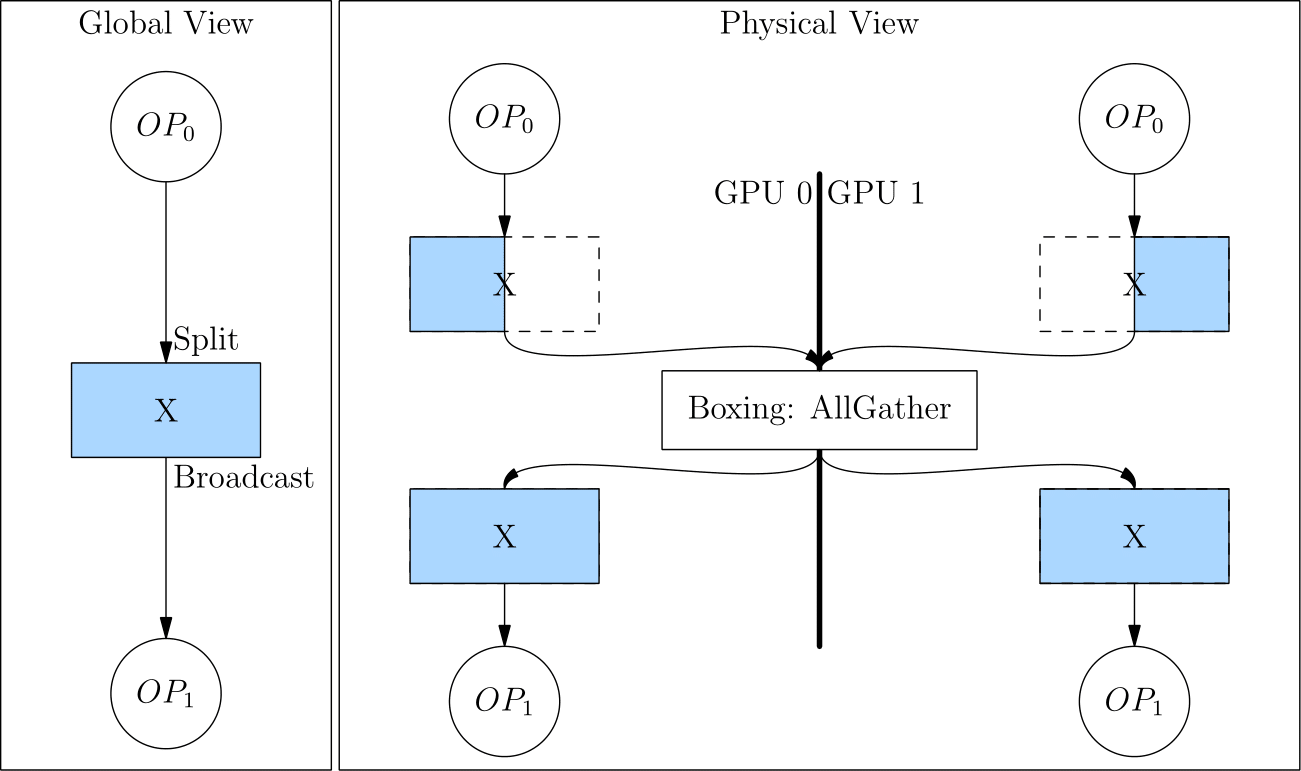# Global Tensor¶

## 创建 Global Tensor¶

Note

export MASTER_ADDR=127.0.0.1 MASTER_PORT=17789 WORLD_SIZE=2 RANK=0 LOCAL_RANK=0
python3

export MASTER_ADDR=127.0.0.1 MASTER_PORT=17789 WORLD_SIZE=2 RANK=1 LOCAL_RANK=1
python3


### 直接创建 global tensor¶

• "cuda" 表示在 GPU 设备上。
• placement 的第二个参数是一个 list，集群中的设备会被自动编号，通过数字指定使用哪些设备。[0,1] 表示 global tensor 使用第 0、1 张显卡上。
import oneflow as flow

placement = flow.placement("cuda", [0,1])
sbp = flow.sbp.split(0)
x = flow.randn(4,5,placement=placement, sbp=sbp)
x.shape

import oneflow as flow

placement = flow.placement("cuda", [0,1])
sbp = flow.sbp.split(0)
x = flow.randn(4,5,placement=placement, sbp=sbp)
x.shape


oneflow.Size([4, 5])

oneflow.Size([4, 5])


### 由 global tensor 得到 local tensor¶

x.to_local()
tensor([[ 2.9186e-01, -3.9442e-01,  4.7072e-04, -3.2216e-01,  1.7788e-01],
[-4.5284e-01,  1.2361e-01, -3.5962e-01,  2.6651e-01,  1.2951e+00]],
device='cuda:0', dtype=oneflow.float32)

x.to_local()
tensor([[-0.4363,  0.9985, -2.5387,  0.3003,  0.3803],
[ 0.0556, -0.8077,  1.1191, -2.1278,  0.1468]], device='cuda:1',
dtype=oneflow.float32)


### 由 local tensor 转换得到 global tensor¶

import oneflow as flow

x = flow.randn(2,5)
placement = flow.placement("cuda", [0,1])
sbp = flow.sbp.split(0)
x_global = x.to_global(placement=placement, sbp=sbp)
x_global.shape

import oneflow as flow

x = flow.randn(2,5)
placement = flow.placement("cuda", [0,1])
sbp = flow.sbp.split(0)
x_global = x.to_global(placement=placement, sbp=sbp)
x_global.shape


## 实践 SBP Signature 的作用¶

### 数据并行¶import oneflow as flow

placement = flow.placement("cuda", [0,1])
x = flow.randn(4,5,placement=placement, sbp=flow.sbp.split(0))
y = flow.matmul(x,w)
y.sbp
y.shape

import oneflow as flow

placement = flow.placement("cuda", [0,1])
x = flow.randn(4,5,placement=placement, sbp=flow.sbp.split(0))
y = flow.matmul(x,w)
y.sbp
y.shape


(oneflow.sbp.split(dim=0),)
oneflow.Size([4, 8])

(oneflow.sbp.split(dim=0),)
oneflow.Size([4, 8])


### 模型并行¶import oneflow as flow

placement = flow.placement("cuda", [0,1])
w = flow.randn(5,8,placement=placement, sbp=flow.sbp.split(1))
y = flow.matmul(x,w)
y.sbp
y.shape

import oneflow as flow

placement = flow.placement("cuda", [0,1])
w = flow.randn(5,8,placement=placement, sbp=flow.sbp.split(1))
y = flow.matmul(x,w)
y.sbp
y.shape


(oneflow.sbp.split(dim=1),)
oneflow.Size([4, 8])

(oneflow.sbp.split(dim=1),)
oneflow.Size([4, 8])


## 扩展阅读¶

### 多机训练时的环境变量¶

• MASTER_ADDR：多机训练的第0号机器的 IP
• MASTER_PORT：多机训练的第0号机器的监听端口，不与已经占用的端口冲突即可
• WORLD_SIZE：整个集群中计算设备的数目，因为目前还不支持各个机器上显卡数目不一致，因此 WORLD_SIZE 的数目实际上是 $$机器数目 \times 每台机器上的显卡数目$$。如我们这个例子中，是单机2卡的情况，因此 WORLD_SIZE=2

RANKLOCAL_RANK 都是对计算设备的编号，不同的是 RANK 是“全局视角”的编号，LOCAL_RANK 某个特定机器上的“局部视角”的编号。当是单机训练（单机单卡或单机多卡）时，两者是没有区别的。以上的例子中，有两个显卡，分别是0号和1号。

RANK LOCAL_RANK

### Boxing（自动转换 SBP）¶split(1) 转换为 broadcast，相当于做了一次 AllGather 操作。如下图所示。